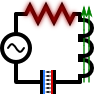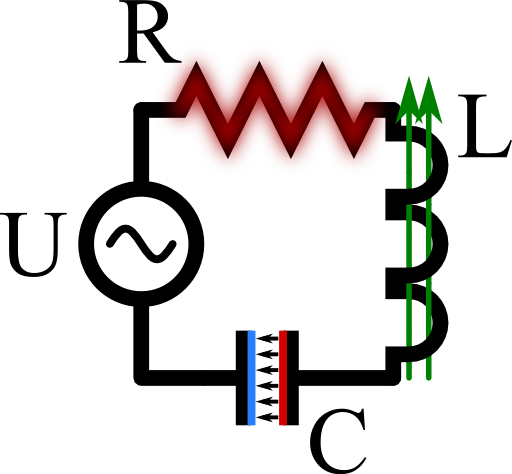Dissipation in the Serial RLC CircuitWe will study one of the main problems of circuit theory and find basic concepts. Find out when the time-averaged dissipated power in the circuit is maximal using the phasor notation. Get to know a method to calculate the complex eigenfrequencies of the circuit.

Problem StatementCalculate the time-averaged dissipated power $$\left\langle P\left(t\right)\right\rangle =\frac{1}{2\tau}\int_{t-\tau}^{t+\tau}P\left(t\right)dt$$ at the ohmic resistor $$R$$ depending on the driving frequency $$\omega_{0}$$ of the voltage source, $$U\left(t\right)=U_{0}\exp\left(-\mathrm{i}\omega_{0}t\right)$$. For which frequency is the dissipation maximal and, physically, why?

Now short the power source and find which relation the impedances have to obey. This relation can also be read as a condition for the complex so-called eigenfrequencies $$\omega_{i}$$ at which the shortened circuit has to oscillate. Determine the $$\omega_{i}$$  and explain their meaning.